Updating search results...

# 55 Results

View
Selected filters:
• numbers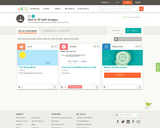Conditional Remix & Share Permitted
CC BY-NC-SA
Rating
0.0 stars

This course features a video review of addition up to the sum of ten for first graders.

Subject:
Mathematics
Material Type:
Lecture
Provider:
CK-12 Foundation
Provider Set:
CK-12 Elementary Math
06/06/2019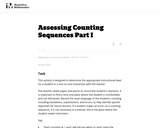Unrestricted Use
CC BY
Rating
0.0 stars

This activity is designed to determine the appropriate instructional level for a student in a one-on-one interaction with the teacher.

Subject:
Mathematics
Material Type:
Activity/Lab
Provider:
Illustrative Mathematics
Provider Set:
Illustrative Mathematics
Author:
Illustrative Mathematics
05/01/2012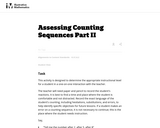Unrestricted Use
CC BY
Rating
0.0 stars

This activity is designed to determine the appropriate instructional level for a student in a one-on-one interaction with the teacher.

Subject:
Mathematics
Material Type:
Activity/Lab
Provider:
Illustrative Mathematics
Provider Set:
Illustrative Mathematics
Author:
Illustrative Mathematics
05/01/2012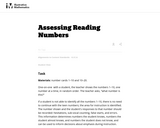Unrestricted Use
CC BY
Rating
0.0 stars

In this assessment in a one-to-one setting, a student is shown the numbers from 1Đ10, one number at a time, in random order. The teacher asks, Ňwhat number is this?"

Subject:
Mathematics
Material Type:
Activity/Lab
Provider:
Illustrative Mathematics
Provider Set:
Illustrative Mathematics
Author:
Illustrative Mathematics
05/01/2012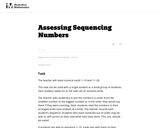Unrestricted Use
CC BY
Rating
0.0 stars

This assessment task can be used with a single student or a small group of students. Each student needs his or her own set of numeral cards.

Subject:
Mathematics
Material Type:
Activity/Lab
Provider:
Illustrative Mathematics
Provider Set:
Illustrative Mathematics
Author:
Illustrative Mathematics
05/01/2012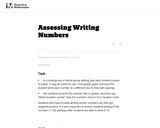Unrestricted Use
CC BY
Rating
0.0 stars

This assessment may be used in a small group or whole group setting, give each student a piece of paper. Students who have trouble writing certain numbers can then get targeted practice.

Subject:
Mathematics
Material Type:
Activity/Lab
Provider:
Illustrative Mathematics
Provider Set:
Illustrative Mathematics
Author:
Illustrative Mathematics
05/01/2012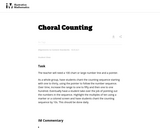Unrestricted Use
CC BY
Rating
0.0 stars

This task suggests methods of introducing and continuing choral counting in the classroom.

Subject:
Mathematics
Material Type:
Activity/Lab
Provider:
Illustrative Mathematics
Provider Set:
Illustrative Mathematics
Author:
Illustrative Mathematics
05/01/2012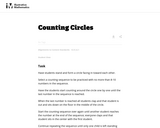Unrestricted Use
CC BY
Rating
0.0 stars

This task suggests ways to incorporate counting circles into classroom activities.

Subject:
Mathematics
Material Type:
Activity/Lab
Provider:
Illustrative Mathematics
Provider Set:
Illustrative Mathematics
Author:
Illustrative Mathematics
05/01/2012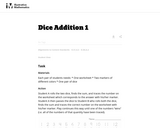Unrestricted Use
CC BY
Rating
0.0 stars

This task supports students in correctly writing numbers. Because students have to trace the number, instead of coloring in a bubble with the number in it or circling the correct number, they gain handwriting practice as well as counting and addition practice.

Subject:
Mathematics
Material Type:
Activity/Lab
Provider:
Illustrative Mathematics
Provider Set:
Illustrative Mathematics
Author:
Illustrative Mathematics
11/06/2012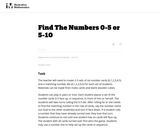Unrestricted Use
CC BY
Rating
0.0 stars

This card game allows students to practice number skills.

Subject:
Mathematics
Material Type:
Activity/Lab
Provider:
Illustrative Mathematics
Provider Set:
Illustrative Mathematics
Author:
Illustrative Mathematics
05/01/2012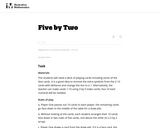Unrestricted Use
CC BY
Rating
0.0 stars

This game will reinforce number before and after as well as reading and sequencing numbers.

Subject:
Mathematics
Material Type:
Activity/Lab
Provider:
Illustrative Mathematics
Provider Set:
Illustrative Mathematics
Author:
Illustrative Mathematics
05/01/2012Rating
0.0 stars

In this activity, students learn the numbers from 1 to 15 in Spanish by firing the correct number of meatballs onto a plate of spaghetti.

Subject:
Spanish
World Languages
Material Type:
Activity/Lab
Provider:
Universal Studios Home Entertainment Family Productions
Author:
Universal Studios Home Entertainment Family Productions
02/26/2019Conditional Remix & Share Permitted
CC BY-NC
Rating
0.0 stars
Rating
0.0 stars
Subject:
Mathematics
Material Type:
Full Course
Provider:
Pearson
11/02/2020Conditional Remix & Share Permitted
CC BY-NC
Rating
0.0 stars

Rational Numbers

Type of Unit: Concept

Prior Knowledge

Students should be able to:

Solve problems with positive rational numbers.
Plot positive rational numbers on a number line.
Understand the equal sign.
Use the greater than and less than symbols with positive numbers (not variables) and understand their relative positions on a number line.
Recognize the first quadrant of the coordinate plane.

Lesson Flow

The first part of this unit builds on the prerequisite skills needed to develop the concept of negative numbers, the opposites of numbers, and absolute value. The unit starts with a real-world application that uses negative numbers so that students understand the need for them. The unit then introduces the idea of the opposite of a number and its absolute value and compares the difference in the definitions. The number line and positions of numbers on the number line is at the heart of the unit, including comparing positions with less than or greater than symbols.

The second part of the unit deals with the coordinate plane and extends student knowledge to all four quadrants. Students graph geometric figures on the coordinate plane and do initial calculations of distances that are a straight line. Students conclude the unit by investigating the reflections of figures across the x- and y-axes on the coordinate plane.

Subject:
Mathematics
Provider:
PearsonConditional Remix & Share Permitted
CC BY-NC
Rating
0.0 stars

Students analyze whether given statements are possible or impossible using their definitions of absolute value and the opposite of a number. If the statements are possible, students give an example of a pair of numbers that fit the statement. If the statements are impossible, students explain why.Key ConceptsA number and the opposite of the number always have the same absolute value.In general, taking the opposite of n changes the sign of n. For example, the opposite of 3 is −3.In general, taking the absolute value of n gives a number |n|, which is always positive. For example, |3| = 3 and |−3| = 3.Since the opposite of 0 is 0 (which is neither positive nor negative), therefore −0 = 0. The number 0 is the only number which is its own opposite.Goals and Learning ObjectivesFind pairs of numbers that satisfy different statements about absolute values and/or the opposites of numbers.State when it is impossible to find a pair of numbers that satisfies the statement and explain why.

Material Type:
Lesson Plan
Author:
Pearson
11/02/2020Conditional Remix & Share Permitted
CC BY-NC
Rating
0.0 stars

Ratios

Type of Unit: Concept

Prior Knowledge

Students should be able to:

Calculate with whole numbers up to 100 using all four operations.
Understand fraction notation and percents and translate among fractions, decimal numbers, and percents.
Interpret and use a number line.
Use tables to solve problems.
Use tape diagrams to solve problems.
Sketch and interpret graphs.
Write and interpret equations.

Lesson Flow

The first part of the unit begins with an exploration activity that focuses on a ratio as a way to compare the amount of egg and the amount of flour in a mixture. The context motivates a specific understanding of the use of, and need for, ratios as a way of making comparisons between quantities. Following this lesson, the usefulness of ratios in comparing quantities is developed in more detail, including a contrast to using subtraction to find differences. Students learn to interpret and express ratios as fractions, as decimal numbers, in a:b form, in words, and as data; they also learn to identify equivalent ratios.

The focus of the middle part of the unit is on the tools used to represent ratio relationships and on simplifying and comparing ratios. Students learn to use tape diagrams first, then double number lines, and finally ratio tables and graphs. As these tools are introduced, students use them in problem-solving contexts to solve ratio problems, including an investigation of glide ratios. Students are asked to make connections and distinctions among these forms of representation throughout these lessons. Students also choose a ratio project in this part of the unit (Lesson 8).

The third and last part of the unit covers understanding percents, including those greater than 100%.

Students have ample opportunities to check, deepen, and apply their understanding of ratios, including percents, with the selection of problems in the Gallery.

Subject:
Mathematics
Statistics and Probability
Provider:
PearsonConditional Remix & Share Permitted
CC BY-NC
Rating
0.0 stars

This lesson formally introduces and defines a ratio as a way of comparing numbers to one another.Key ConceptsA ratio is defined by the following characteristics:A ratio is a pair of numbers (a:b).Ratios are used to compare two numbers.The value of a ratio a:b is the quotient a ÷ b, or the result of dividing a by b.Other important features of ratios include the following:A ratio does not always tell you the values of quantities being compared.The order of values in a ratio matters.Goals and Learning ObjectivesIntroduce a formal definition of ratio.Use the definition of ratio to solve problems related to comparing quantities.Understand that ratios do not always tell you the values of the quantities being compared.Understand that the order of values in a ratio matters.

Material Type:
Lesson Plan
Author:
Pearson
11/02/2020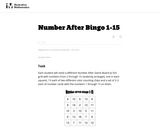Unrestricted Use
CC BY
Rating
0.0 stars

This number after bingo activity increases student flexibility with the number sequence and their ability to start counting sequences at various points.

Subject:
Mathematics
Material Type:
Activity/Lab
Provider:
Illustrative Mathematics
Provider Set:
Illustrative Mathematics
Author:
Illustrative Mathematics
05/01/2012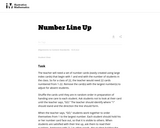Unrestricted Use
CC BY
Rating
0.0 stars

This number line up activitiy gets students out of their seats and helps reinforce the counting sequence.

Subject:
Mathematics
Material Type:
Activity/Lab
Provider:
Illustrative Mathematics
Provider Set:
Illustrative Mathematics
Author:
Illustrative Mathematics
05/01/2012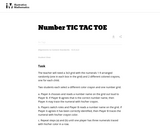Unrestricted Use
CC BY
Rating
0.0 stars

This activity is played in pairs and gives students practice reading numbers out loud and tracing them.

Subject:
Mathematics
Material Type:
Activity/Lab
Provider:
Illustrative Mathematics
Provider Set:
Illustrative Mathematics
Author:
Illustrative Mathematics